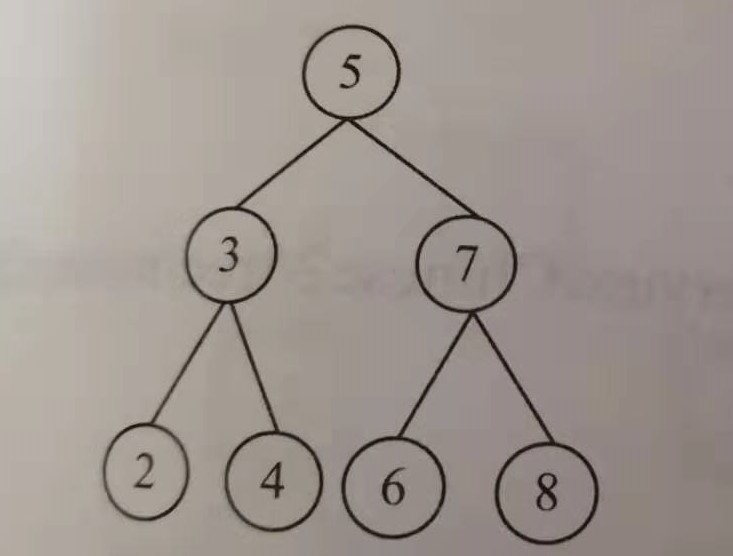## Tencent back-end intern development interview cool: the k-th largest node in the binary search tree

This is my first interview. I haven't seen the interview before or brushed the oj. The result is quite unexpected. Now I will record this question in memory of my lost job opportunity in the goose farm.

Question:
Given a binary search tree, please find the k-th largest node in it. For example, in the figure below, the value of the third largest node is 4.First of all, what is a binary search tree?

The definition of Baidu Encyclopedia: Binary search tree is either an empty tree, or a binary tree with the following properties: if its left subtree is not empty, the value of all nodes on the left subtree is less than its The value of the root node, if its right subtree is not empty, then the values ​​of all nodes on its right subtree are greater than the value of its root node, and its left and right subtrees are also binary sorted trees respectively.

If it is a binary sort tree, a very important property is that the in-order traversal of the binary sort tree is an ascending sequence of numbers.
When I was interviewing, I didn't even know what to do.

Then, to find the k-th largest node in the binary sorting tree, you only need to traverse the binary tree in order, then you can find the k-th largest node in the binary tree.

``````public class TreeNode {

public int val;
public TreeNode left;
public TreeNode right;

public TreeNode(int x) {

val = x;
}
}

public class 二叉搜索树的第k大节点 {

private static int mK;

public static TreeNode kThNode(TreeNode root, int k) {

if (root == null || k == 0) {

return null;
}
mK = k;
return findKthNode(root);
}

/**
* 使用递归的方式进行求解
*
* @param root 根节点
* @return 第k大节点
*/
private static TreeNode findKthNode(TreeNode root) {

TreeNode target = null;

// 左子树不为空的时候递归寻找
if (root.left != null) {

target = findKthNode(root.left);
}
// 当没有找到目标的时候，我们需要对k-1
if (target == null) {

if (mK == 1) {

return root;
}
mK--;
}

// 没有找到目标并且右子树不为空的时候，递归地在右子树中寻找
if (target == null && root.right != null) {

target = findKthNode(root.right);
}
return target;
}

/**
* 使用非递归的方式进行求解
*
* @param root 根节点
* @param k    k表示第k大元素
*/
public static void findKthNumber(TreeNode root, int k) {

TreeNode temp = root;
int result = 0;
Stack<TreeNode> stack = new Stack<>();
while (!stack.isEmpty() || temp != null) {

// 沿着左子树走到尽头
while (temp != null) {

stack.push(temp);
temp = temp.left;
}
// 左分支走到尽头，开始遍历右分支
if (!stack.isEmpty()) {

temp = stack.pop();
result++;
if (result == k) {

System.out.print(temp.val + " ");
return;
}
temp = temp.right;
}
}
}

public static void main(String[] args) {

TreeNode root=new TreeNode(5);
TreeNode node3=new TreeNode(3);
TreeNode node2=new TreeNode(2);
TreeNode node4=new TreeNode(4);
root.left=node3;
node3.left=node2;
node3.right=node4;
System.out.println(kThNode(root,3).val); //输出4
}
}

``````

After I did it, I found it was not difficult

### Guess you like

Origin blog.csdn.net/liu_12345_liu/article/details/103113182
Recommended
Ranking
Daily